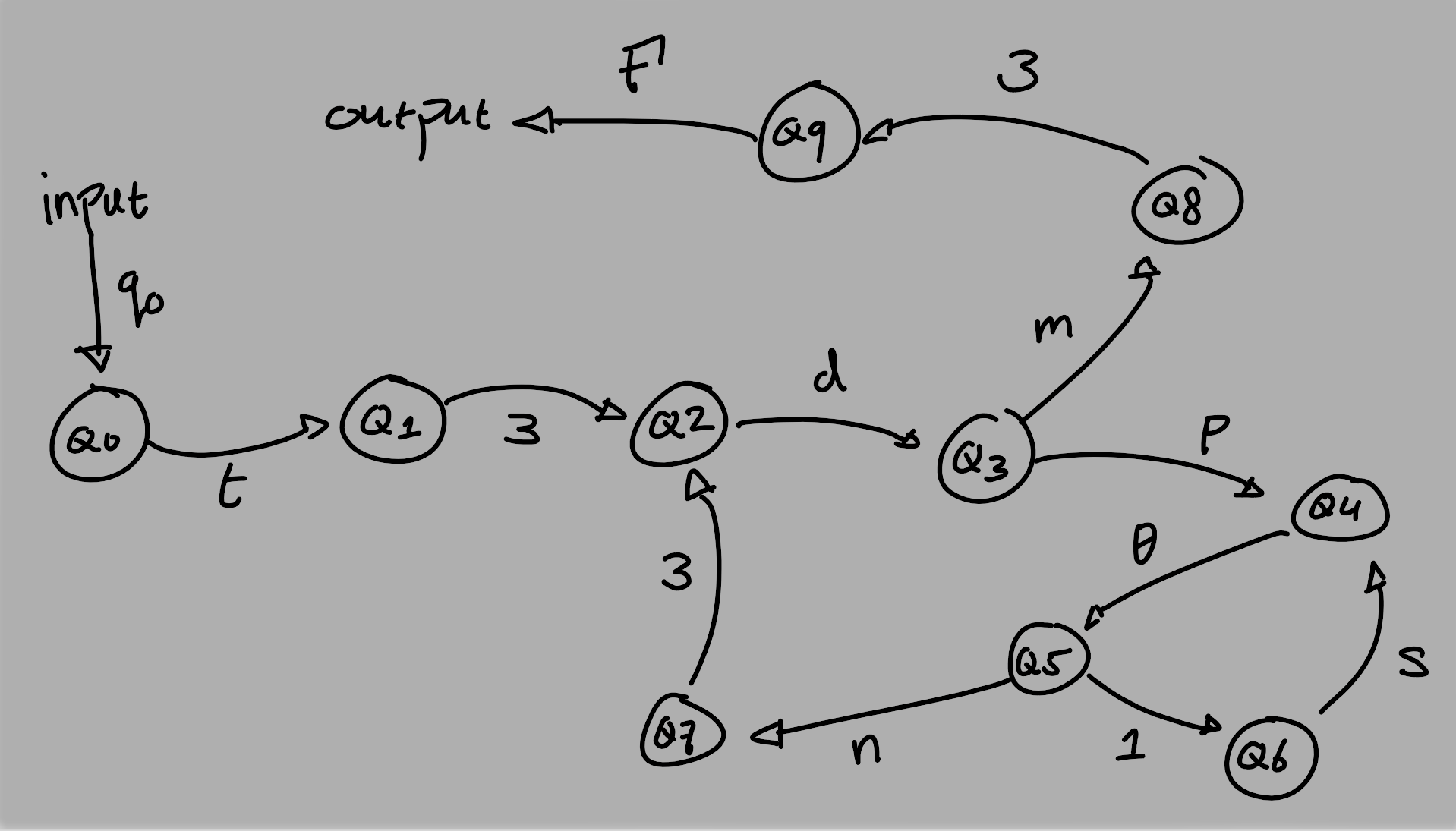# NetOn CTF 2021 - MathTomata

Misc – 245 pts (9 solves) – Chall author: Nikeley

A finite-state machine construction with a solution that itself is the flag.

## ChallengeSo a ‘DFA’, or Deterministic Finite Automaton, is a kind of finite-state machine. This means we can put in some input and out comes something depending on its structure. A quick read on Wikipedia explain enough in order to figure this puzzle out. We are given the following information about our DFA

Parameter 		Meaning						Value
------------------------------------------------------------------
Q 				States / nodes				Q0 through Q9
Σ				Possible messages			{d,m,n,p,s,t,0,1,3}
δ 				Transition function 		_see Table below_
q0 				Initial state 				Q0
F 				Accept / final state 		Q9

  δ	 |	d 	m 	n 	p 	s 	t 	0 	1 	3
------------------------------------------------------------------
Q0  |						Q1
Q1  |									Q2
Q2  |	Q3
Q3  |		Q8		Q4
Q4  | 							Q5
Q5  | 			Q7 					Q6
Q6  | 					Q4
Q7  | 									Q2
Q8  | 									Q9
Q9  |


## Solution

In other words, we can picture this as a collection of 10 nodes, or stepping stones, that are connected to each other according to the transition function. For every step we take, we pick up one of the possible messages in order to string our flag together. Furthermore, we need to start at Q0, end at Q9, and take exactly 13 steps (as denoted in the challenge description). A sketch of this system is given below.Following the stepping stones, we can derive the following flag

NETON{t3dp01s0n3dm3}


F. o7